How Cheenta works to ensure student success?
Explore the Back-Story

# Roots of cubic equation | AMC-10A, 2010 | Problem 21Try this beautiful problem from Algebra based Roots of the cubic equation.

## Roots of cubic equation - AMC-10A, 2010- Problem 21

The polynomial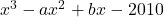has three positive integer roots. What is the smallest possible value of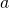?

••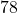•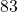•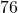•### Key Concepts

Algebra

Vieta's Relation

roots of the equation

Answer:AMC-10A (2010) Problem 19

Pre College Mathematics

## Try with Hints

The given equation is.we have to find out the smallest possible value of.From Vieta's Relation we know that if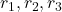are the roots of equation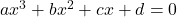then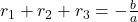and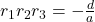can you finish the problem........

Thereforeis the sum of the three roots of the polynomial. andis the product of the three integer roots.Now the factors of=.there are only three roots to the polynomial so out of four roots we have to choose three roots such that two of the four prime factors must be multiplied so that we are left with three roots. To minimize,and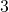should be multiplied,

can you finish the problem........

Therefore the value of=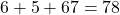## Subscribe to Cheenta at Youtube

Try this beautiful problem from Algebra based Roots of the cubic equation.

## Roots of cubic equation - AMC-10A, 2010- Problem 21

The polynomialhas three positive integer roots. What is the smallest possible value of?

•••••### Key Concepts

Algebra

Vieta's Relation

roots of the equation

Answer:AMC-10A (2010) Problem 19

Pre College Mathematics

## Try with Hints

The given equation is.we have to find out the smallest possible value of.From Vieta's Relation we know that ifare the roots of equationthenandcan you finish the problem........

Thereforeis the sum of the three roots of the polynomial. andis the product of the three integer roots.Now the factors of=.there are only three roots to the polynomial so out of four roots we have to choose three roots such that two of the four prime factors must be multiplied so that we are left with three roots. To minimize,andshould be multiplied,

can you finish the problem........

Therefore the value of=## Subscribe to Cheenta at Youtube

This site uses Akismet to reduce spam. Learn how your comment data is processed.

### Knowledge Partner